# Chi-Square Table

10K+Everyone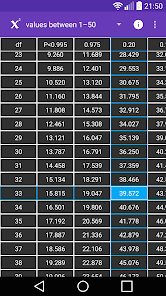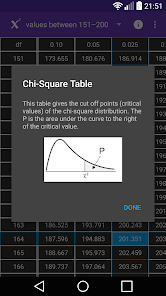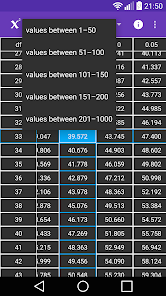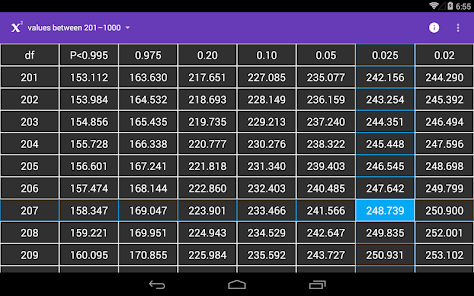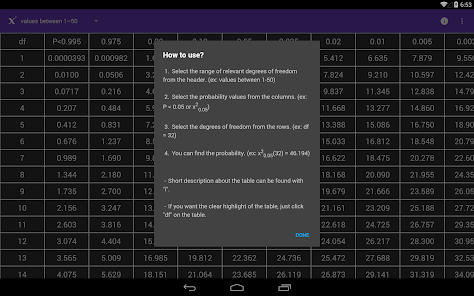"Chi-Square Table" application is developed to provide easy and accurate information to users. You can find all Chi-Square critical values on a single app and easy to use table.

The Chi-Square Table application can be benefited in quality concerns and statistic lessons. It includes basic explanations and the Chi-Square Table in a usable format.

You can easily download and start to use Chi-Square Table application, you don’t have to carry Chi-Square Table or need internet connection anymore.

In probability theory and statistics, the chi-squared distribution (also chi-square or x²-distribution) with k degrees of freedom is the distribution of a sum of the squares of k independent standard normal random variables. The chi-squared distribution is used in the common chi-squared tests for goodness of fit of an observed distribution to a theoretical one, the independence of two criteria of classification of qualitative data, and in confidence interval estimation for a population standard deviation of a normal distribution from a sample standard deviation.
Updated on
May 27, 2020

## Data safety